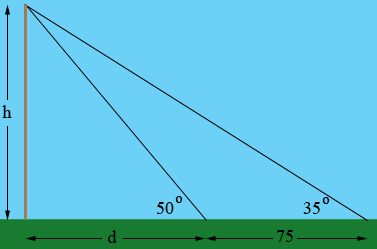SEARCH HOMEMath Central Quandaries & QueriesQuestion from Grail, a student: at a certain point the angle of elevation of the top of a flagpole which stands on a level ground is 35 degree. 75 ft. nearer the pole, the angle of elevation is 50 degree. How high is the poleHi Grail,

The key here is to draw a diagram.There are two right triangles here.

What trig function relates h, d and the angle with measure 50 degrees? This gives you an equation involving d and h.

What trig function relates h, d + 75 and the angle with measure 35 degrees? This gives you another equation involving d and h.

Solve the equations for h.

PennyMath Central is supported by the University of Regina and The Pacific Institute for the Mathematical Sciences.